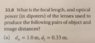# Optics focal length and optical power Question

crystal1001
Homework Statement:
What is the focal length and optical power (in diopters) of the lenses used to produce the following pairs of object and image distances?

do = 1.0 m
di = 0.33 m
Relevant Equations:
focal length: 1/f = 1/do + 1/di
Power (diopters): 1/fD
1/f = 1/dₒ + 1/dᵢ = 1/1.0 + 1/0.33 = 4.03 m (focal length)

Power: 1/4.03 m or 0.248 (0.25)

*around to the2 decimal points? or leave it as a fraction?

((Did I do this correctly? I am not sure if this is correct and is it okay to be in meters not centimeters?))

#### Attachments

•20191118_221442.jpg
37.4 KB · Views: 143
•Delta2

Gold Member
Looks Ok to me!on this part. See next post by @ehild. Sorry.

The original numbers were 2 significant figures, so the answer should have the same, which you have. The same goes for the units, you matched what was given. 👍

Last edited:
Homework Helper
do = 1.0 m
di = 0.33 m
Homework Equations: focal length: 1/f = 1/do + 1/di
Power (diopters): 1/fD

1/f = 1/dₒ + 1/dᵢ = 1/1.0 + 1/0.33 = 4.03 m (focal length)
The focal length is f, not 1/f. 1/f is the power (diopters) if f is measured in meters.

•Tom.G